• Post Author:

#### Classification of organic compounds ( Hydrocarbons)

Organic compounds are defined as the hydrocarbons (compounds containing carbon and hydrogen) and their derivatives in which covalently bonded carbon is an essential constituent.

Organic compounds are classified as :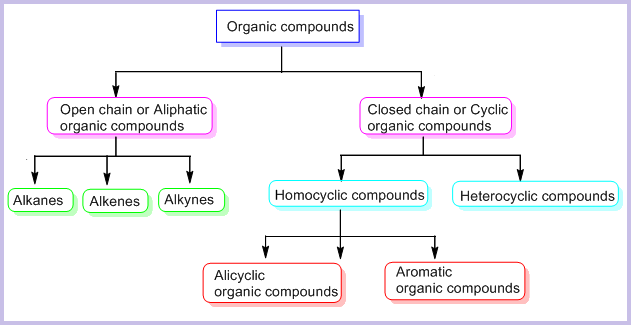1. Open chain organic compounds :

Organic compounds in which the terminal C-atoms are not joined together are called open chain compounds. Eg.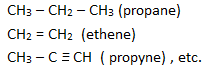The open chain organic compounds can be further classified as,

Alkanes : Alkanes are the saturated hydrocarbons with general formula CnH2n+2. They contain only carbon-carbon and carbon-hydrogen single bonds in their molecules. For example: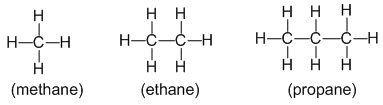• Alkanes are also called paraffins (because they have a little affinity towards a general reagent. In other words, alkanes are less reactive substances. They undergo reactions under drastic conditions)

Note : Alkanes are called saturated because all the possible sites (i.e. 4) are bonded with other atoms.

But in alkenes and alkynes there is a possibility of addition of atoms or groups so they are called unsaturated.

Alkenes : Alkenes are unsaturated hydrocarbons with general formula CnH2n. They contain at least one carbon to carbon double bond in their molecules. For example: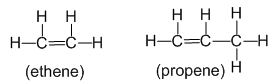• Alkenes are also called olefins ( i.e. oil forming because they form oily liquids on reaction with chlorine gas.

Alkynes : Alkynes are unsaturated hydrocarbons with general formula CnH2n-2. They contain at least one carbon to carbon triple bond in their molecules. For example: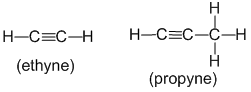2. Closed chain (cyclic) organic compounds :

Organic compounds in which the terminal carbons are joined together to form a cyclic structure are called closed chain or cyclic organic compounds. For exampne: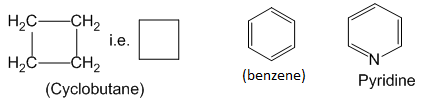Cyclic organic compounds are further classified as – homocyclic and heterocyclic organic compounds.

Homocyclic compounds : Cyclic organic compounds in which the ring forming atoms are only carbon are called homocyclic or more specifically carbocyclic compounds.

Homocyclic compounds can be further classified as – Alicyclic and Aromatic compounds.

Alicyclic compounds :

Closed chain organic compounds whose properties are similar to open chain aliphatic compounds are called alicyclic compounds. For example: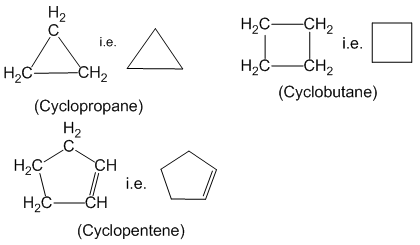Aromatic compounds :

Benzene and those cyclic compounds that chemically behave as benzene are called aromatic compounds. For example: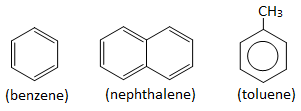Aromatic compounds obey Huckel’s rule.

Note : Huckel’s rule :

Huckel’s rule states that a cyclic and planar molecule is aromatic if it contains 4n+2 delocalized π electrons, where n = 0,1,2,3,4,etc.

Examples : Benzene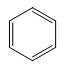Benzene is cyclic and planar and has cyclic overlap of p-orbitals. There are 3 double bonds i.e. 6 delocalized π – electrons, which is consistant with Huckel’s rule.

i.e. 4n+2 = 6

4n= 4

n = 1(which is an integer)

Therefore, benzene is an aromatic compound. It will show aromaticity.

Heterocyclic compounds :

Cyclic organic compounds in which at least one heteroatom (i.e. atom other than carbon eg. N, O or S ) is present as one of the ring forming atoms are called heterocyclic compounds. Examples :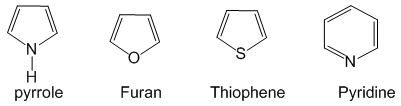#### Formula of organic compounds

Molecular formula :

It represents actual number of atoms of all the elements present in one molecule of the compound. For example:

methane = CH4

ethane = C2H6

ethene = C2H4

benzene = C6H6 , etc.

Empirical formula :

It represents simple whole number ratio of atoms of all the elements in one molecule of the compound. For example:

ethane = CH3

ethene = CH2

benzene = CH , etc.

Electron-dot formula : In this formula valence electrons are represented by dots placed around the chemical symbol. It is also called Lewis formula. For example: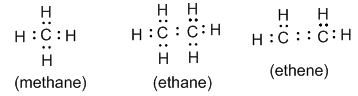Structural formula : It indicates how the atoms are bonded in a molecule of the compound. For example: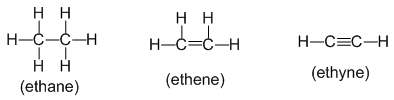Contracted or condensed formula :

It is the structural formula in contracted form to save space and time.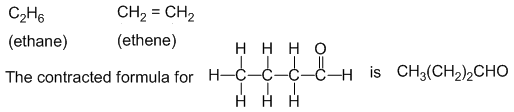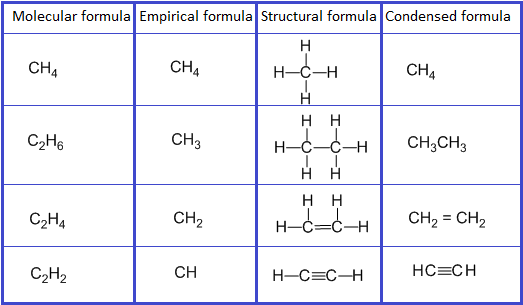Bond – line formula :

In this type of formula, carbon and hydrogen atoms are not shown and only hetero atoms are shown. The point of intersection represents carbon along with required number of hydrogen to satisfy the valency of carbon. For example: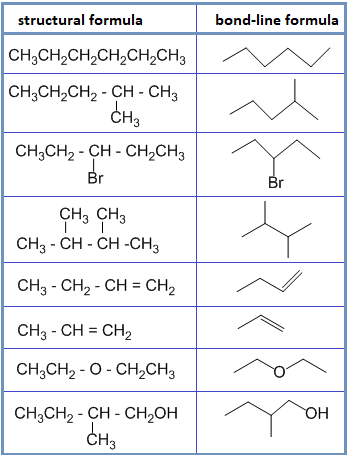Spatial formula :

This formula represents the three dimensional shape or arrangement of atoms in the molecule. For example :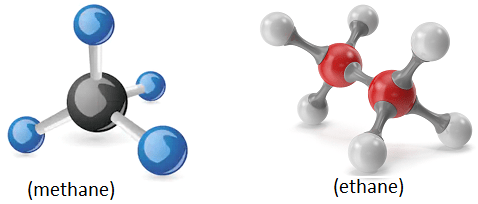#### Functional group

An atom or group of atoms in a molecule which largely determines the chemical properties of the organic compounds is known as functional group. All the compounds having same functional group show similar properties and constitute a class or a family.

For example: organic compounds having – OH as functional group constitutes a class of compounds called alcohol.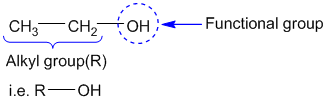Some other examples of functional group are :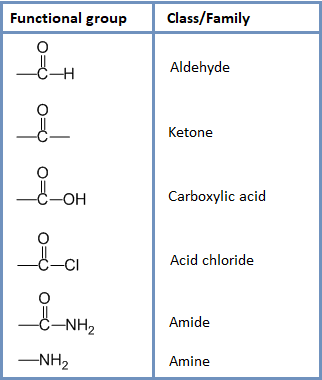#### Homologous series

The series of organic compounds having same general formula and similar chemical properties but different physical properties in which one member differs from other member by single – CH2 unit is known as homologous series. For example: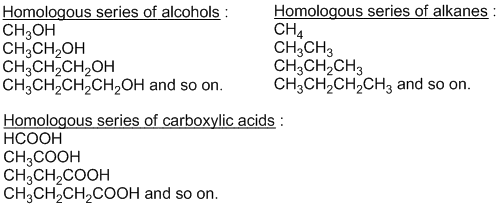Each member of homologous series is called homologue and phenomenon of making homologous series is called homology.

Characteristics of homologous series :

• All the members of homologous series have same functional group.
• All the members of homologpous series have same chemical properties.
• All the members of homologous series can be prepared by a common method of preparation. Eg.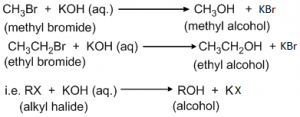• All the members of homologous series can be represented by same general formula. Eg.

CnH2n+2 = Alkane

CnH2n = Alkene

CnH2n-2 = Alkyne

CnH2n+1OH = Alcohol, etc.

• Their molecular masses increases gradually hence their physical properties (eg. melting point and boiling point) changes gradually.
• Each member differs from the adjacent member by methylene (-CH2-) unit.

Q) Write down the 1st, IInd, IIIrd and IVth homologue of aldehyde homologous series.

Ist homologue = HCHO

IInd homologue = CH3CHO

IIIrd homologue = CH3CH2CHO

IVth homologue = CH3CH2CH2CHO

See the IUPAC Nomenclature of Organic compounds ……

Also see the Isomerism

#### Objective questions

1. Cyclic organic compounds possessing the properties of aliphatic compounds are called ___ compounds.

a. Aromatic        c. Carbocyclic

b. Homocyclic    d. Alicyclic

2. The formula which represents the simple whole number ratio of different atoms present in one molecule of a compound is known as :

a. Molecular formula   c. Condensed formula

b. Empirical formula    d. Electron dot formula

3. An organic compound has empirical formula CH2O and molecular weight 90. It’s molecular formula will be :

a. C6H12O6    c. C2H4O2

b. C3H6O3      d. C3H9O6

4. General formula of an alkene is :

a. CnH2n       c. CnH2n-2

b. CnH2n+2    d. CnH2n-1

5. A hydrocarbon is found to contain 81.80% carbon and 18.20% hydrogen. It’s empirical formula will be :

a. C4H8    c. C3H8

b. C2H6    d. C3H6

6. If two compounds have same empirical formula but different molecular formula, they must have :

a. Different percentage composition

b. Different molecular weight

c. Same viscosity

d. Same vapour density

7. Which of the following is an aromatic compound :

a. Benzene hexachloride   c. Cyclobutane

b. Cyclohexane     d. Toluene

8. Which of the following is a heterocyclic aromatic compound :

a. Nephthalene     c. Furan

b. Benzene hexachloride   d. Toluene

9. Which of the following belongs to a homologous series ?

a. Methanol, Ethanol, ethanoic acid

b. Propane, Propene, propyne

c. Butane, 2-methylpropane, 2-methylbutane

d. Chloroathane, 2-chloropropane, 1-chlorobutane

10. Which of the following is not true about homologous series ?

a. Adjacent members of group differ by one –CH2 group.

b. Adjacent members of group differs by a mass of 14 amu.

c. Members of a group have same chemical and physical properties.

d. Members of a group can be prepared by same general methods.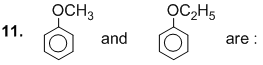a. Isomers    c. allotropes

b. Homologues   d. None

12. Alkanes are also called :

a. Paraffins    c. Acetylene

b. Olefins     d. Both ‘a’ and ‘b’